• 计算数组元素个数方法 int len = sizeof(a)/sizeof(A)  A 是结构体名， a是A的结构体数组名; 定义是 A a[] {x,y,z,}; 计算字符串数组元素个数 int lenth = sizeof(nlanoutput)/sizeof(nlanoutput); ...
计算数组元素个数方法
int len = sizeof(a)/sizeof(A)
A 是结构体名， a是A的结构体数组名; 定义是 A  a[] {x,y,z,};

计算字符串数组元素个数
int lenth = sizeof(nlanoutput)/sizeof(nlanoutput);
nlanoutput 是字符串数组; 定义是 unsigned char nlanoutput[];

展开全文• 我们所说的数组元素个数是指总元素个数 例如： arr中有10元素 arr中有3X4=12元素 那么怎么能求出这个个数给计算机呢？ 对于一维数组 #include <stdio.h> int main() { int arr = { 0 }; ...
我们所说的数组元素个数是指总元素个数
例如：
arr中有10个元素
arr中有3X4=12个元素
那么怎么能求出这个个数给计算机呢？
对于一维数组
#include <stdio.h>

int main()
{
int arr = { 0 };
int sz = sizeof(arr) / sizeof(arr);

printf("%d\n", sz);
return 0;
}

这个时候我们计算出来这个一维数组的元素个数如下：![在这里插入图片描述](https://img-blog.csdnimg.cn/20210124102523489.png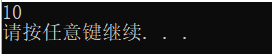对于二维数组
#include <stdio.h>

int main()
{
int arr = { 0 };
int sz = sizeof(arr) / sizeof(arr);

printf("%d\n", sz);
return 0;
}

程序运行结果如下：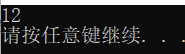此时我们来对上述程序中的sz做一个解释说明。
1.首先我们说对于一维数组，arr代表首元素，对二维数组来说arr表示首元素。
2.其次，数组名表示首元素地址，但有两个例外
（1）sizeof（数组名）时，数组名表示的是整个数组，不是首元素地址，sizeof（数组名）计算的是整个数组的大小。
（2）&数组名时（&表示取地址符），数组名表示整个数组，这里取出的是整个数组的地址。
除此之外，所有的数组名都是首元素地址。
3.最后我们用整个数组元素去除以数组的首元素可以得到数组的元素个数。
感谢阅读。


展开全文• 记录下来，以便日后使用。#include &lt;iostream&gt; using namespace std; int main() { int i; cout &lt;&lt; "sizeof(i) = " &lt;&lt; sizeof(i) &...
记录下来，以便日后使用。#include <iostream>

using namespace std;

int main()
{
int i;
cout << "sizeof(i)  = " << sizeof(i) << endl;
cout << "sizeof(*i) = " << sizeof(*i) << endl;
cout << "sizeof(i) / sizeof(*i) = " << sizeof(i) / sizeof(*i) << endl;
return 0;
}
运行结果如下：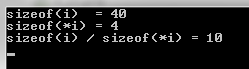其他：#include <iostream>

using namespace std;

int main()
{
int i;
int *p = i;
cout << "sizeof(p)  = " << sizeof(p) << endl;
cout << "sizeof(*p) = " << sizeof(*p) << endl;
cout << "sizeof(p) / sizeof(*p) = " << sizeof(p) / sizeof(*p) << endl;
return 0;
}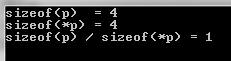#include <iostream>

using namespace std;

int main()
{
int i;
int (*p) = &i;
cout << "sizeof(p)  = " << sizeof(p) << endl;
cout << "sizeof(*p) = " << sizeof(*p) << endl;
cout << "sizeof(p) / sizeof(*p) = " << sizeof(p) / sizeof(*p) << endl;
return 0;
}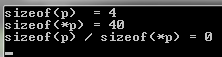展开全文• 一维数组 #include <stdio.h> int main(void) { int arr_one[] = {1,2,3,4,5,6,7,8,9,10}; unsigned long int arr_len = sizeof(arr_one)/sizeof(arr_one); printf("%ld\n",arr_len); ...
通过计算数组的总大小和单个数组元素的大小便可算得数组元素个数。
一维数组
/*test_one.c*/
#include <stdio.h>

int main(void)
{
int arr[] = {1,2,3,4,5,6,7,8,9,10};
unsigned long int arr_len = sizeof(arr)/sizeof(arr);
printf("%ld\n",arr_len);

return 0;
}

/*测试输出*/
10

这个比较简单，sizeof(arr)是全部元素的大小，sizeof(arr)是单个元素的大小。
二维数组
/*test_two.c*/
#include <stdio.h>

int main(void)
{
int arr[] = 	{1,2,3,
4,5,6,
7,8,9,
10};
unsigned long int arr_len1 = sizeof(arr)/sizeof(arr);
unsigned long int arr_len2 = sizeof(arr)/sizeof(arr);
unsigned long int arr_len3 = sizeof(arr)/sizeof(arr);

printf("%ld\n",arr_len1);
printf("%ld\n",arr_len2);
printf("%ld\n",arr_len3); //数组元素总个数

return 0;
}

/*测试输出*/
4
3
12

上面的写法中，arr数组实际上是这样的：
int arr = {1,2,3,
4,5,6,
7,8,9,
10,0,0};

写成这样更好看一点：
int arr = {{1,2,3},
{4,5,6},
{7,8,9},
{10,0,0}};

可以 想象成一维数组的写法：
int a = {1,2,3};
int b = {4,5,6};
int c = {7,8,9};
int d = {10,0,0};

int arr = {a,b,c,d};

arr相当于a， sizeof( arr )是数组全部元素大小，sizeof( arr )就是单个元素的大小了,一相除就得到了总元素的个数。
指针数组
指针数组、数组指针，傻傻分不清，一般我这样子区分：星号*和数组名arr没有被括号（）包裹起来就是指针数组，反之这样的(*arr)[ ]那就是数组指针。其实主要是看结合优先级，*arr[ ]中arr[ ]结合的优先级最高，然后才是和星号*，那么它就相当于一个数组带了一个星号了，指针数组就是存放指针的数组，本质上是数组，只不过存放的不是普通元素，而是指针罢了。
/*test_three.c*/
#include <stdio.h>

int main(void)
{
char *arr[] = {"1","2","3","4","5","6","7","8","9","10"};
unsigned long int arr_len = sizeof(arr)/sizeof(arr);
printf("%ld\n",arr_len);

return 0;
}

/*测试输出*/
10

结构体数组
/*test_four.c*/
#include <stdio.h>

typedef struct test
{
int value;
unsigned char buf;
}TEST;

int main(void)
{
TEST arr;
unsigned long int arr_len1 = sizeof(arr)/sizeof(arr);
unsigned long int arr_len2 = sizeof(arr)/sizeof(TEST);
unsigned long int arr_len3 = sizeof(arr)/sizeof(struct test);

printf("%ld\n",arr_len1);
printf("%ld\n",arr_len2);
printf("%ld\n",arr_len3);

return 0;
}

/*测试输出*/
10
10
10



展开全文C语言
• ## C++求数组元素个数

千次阅读 2020-03-17 10:31:32
我们只需把问题转换为求数组元素a占用空间的大小上，a占用的空间大小很好确定，即sizeof(a)，这就是sizeof(int)的等价条件，所有通过数组名确定元素个数的表达式如下： int l = sizeof(a)/sizeof(a); ....
• ## 求数组元素个数

千次阅读 2017-10-19 09:59:00
sizeof(a)/sizeof(a)可以求得数组a的元素个数
• 宏定义求数组元素个数#define Length(table) (sizeof(table) / sizeof(table))sizeof
• var a = ArrayBuffer('a', 'b', 'c', 'd', 'e', 'a', 'b', 'f', 'g', 'h', 'a', 'b', 'c', 'i', 'j', 'k', 'l', 'a') var aa = cal(a)  for(i &...//一递归的函数，看到后面和前面相同的删除后面...
• * @param valueNum 计算重复个数元素 * */ function isRepeat(result,valueNum){ //判断数组中重复元素的个数 var arr = []; result.sort() for (var i = 0; i &lt; result.length;) { ...
• 如何利用指向数组的指针得到数组元素个数？问题背景：我得到了一元素个数不确定的数组 array={element1,element2……}现在将一指针指向数组 char * pArray ; pArray= array;如果要通过指针处理数组中每元素， ...
• 整个数组所占字节 除以 数组单个元素所占字节 # include <stdio.h> # include <stdlib.h> # include <string.h> int main(void) { int a[] = {1, 5, 7, 0, 10, 7, 3};//int类型在32位操作...C
• 利用C语言可以实现对数组的各种操作，如输入数组元素，输出数组元素、求数组元素平均值、输出数组元素最大值、输出数组元素最小值、查找某数值元素是否存在、给数组元素排序等功能，接下来，我们对这些功能进行一一...算法 数据结构 c语言
• C++中数组可分为堆区的数组和栈区的数组，对于两种数组C++都没有函数可以直接获取数组...堆区的数组不能计算出包含元素个数。 二、栈区的数组 栈区的数组是系统自动分配的。 int arr = { 1,2,3,4,5,6,7,8,9,0 };C++ C
• 问题：请读者编写一表达式确定一个数组元素的个数，目前只知道这数组名为a，它的数据类型等其他信息都在一黑匣子中定义，无从得知答：我们只需把问题转换为求数组元素a占用空间的大小上，a占用的空间...
• Javascript怎么得到数组长度(也就是数组元素个数)? Javascript怎么获取对象的成员个数? 你肯定想到了array.length!? 那么我们来测试一下下面这例子.  var a = [];  a = 50
• 定义了int型二维数组a后,数组元素a前的数组元素个数，叉乘加法java
• Python简单计算数组元素平均值的方法示例 本文实例讲述了Python简单计算数组元素平均值的方法。分享给大家供大家参考，具体如下： Python 环境：Python 2.7.12 x64 IDE ： Wing IDE Professional 5.1.12-1 题目...
• sizeof是C/C++中的一操作符，用于返回传入的数据的长度（字节） 对于一般的元素类型来说，我们通常使用sizeof获取其长度，也习惯使用其获取数据的长度，但是如果对字符数组或者指针进行sizeof操作，往往无法...c++ 字符串 sizeof strlen...